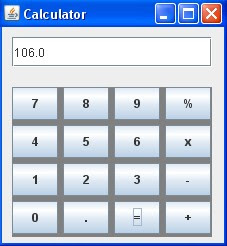### EJB3 | Stateless session bean

To build a statelesss session bean in EJB3, you need to create one interafce and on class. Interface is implemented by class and methods declared in the interaface are available to client for remote access. Bean class and interaface should be public. Bean class is annotated with the annotation @Stateless(mappedName="something") and interafce is annotated with @Remote annotation. Both are compiled and packed in jar file.

Coding for Remote Interface
```package p1;
import javax.ejb.Remote;
@Remote
}
```
Coding for Bean class
```package p1;
import javax.ejb.Stateless;
@Stateless(mappedName="abc")
System.out.println("x="+x+" , y="+y);
int z=x+y;
return z;
}
}
```
Both are compiled and packaged in jar file. you can you following command to package in jar file.
>jar cvf myejb.jar p1
Here p1 is the package in with two class files are produced by compiler.

Building client for statelesss session bean
Client for EJB may be application program, servlet or jsp. At the time of coding of client program, we need to pass server specific coding to Context object. Following program is using the EJB hosted  on weblogic server. So you can see some weblogic specific coding here.
```import javax.naming.*;
import java.util.*;
public static void main(String ar[])throws Exception{
Properties p=new Properties();
p.put(Context.INITIAL_CONTEXT_FACTORY,"weblogic.jndi.WLInitialContextFactory");
p.put(Context.PROVIDER_URL,"t3://localhost:7001");
InitialContext ctx=new InitialContext(p);
System.out.println(x);

}
}
```
Above program will connect to weblogic server and invoke the method add of the EJB having JNDI name abc#p1.AdderIntf and result is received by this program.

### Calculator in java swingimport javax.swing.*;
import java.awt.*;
import java.awt.event.*;
import java.io.*;
class gui1{
static double a=0;
static int op=0;
public static void main(String ar[]){

JFrame f1=new JFrame("Calculator");
f1.setLayout(null);
final JTextField t1=new JTextField(100);
t1.setBounds(10,10,200,30);
JButton b0=new JButton("0");
JButton b1=new JButton("1");
JButton b2=new JButton("2");
JButton b3=new JButton("3");
JButton b4=new JButton("4");
JButton b5=new JButton("5");
JButton b6=new JButton("6");
JButton b7=new JButton("7");
JButton b8=new JButton("8");
JButton b9=new JButton("9");
JButton dot=new JButton(".");
JButton sub=new JButton("-");
JButton equal=new JButton("=");
JButton div=new JButton("%");
JButton mul=new JButton("x");

JPanel buttonPanel=new JPanel();
buttonPanel.setBounds(10,60,200,150);
buttonPanel.setBackground(Color.gray);
//buttonPanel.pack();
buttonPanel.setLayout(new GridLayout(4,4,5,5));

class MyListener1 implements ActionListener{
public void actionPerformed(ActionEvent e){
String cmd=e.getActionCommand();
if(cmd.equals("+")){
a=Double.parseDouble(t1.getText());
op=1;
t1.setText("");
}else if(cmd.equals("-")){
a=Double.parseDouble(t1.getText());
op=2;
t1.setText("");
}else if(cmd.equals("x")){
a=Double.parseDouble(t1.getText());
op=3;
t1.setText("");
}
else if(cmd.equals("%")){
a=Double.parseDouble(t1.getText());
op=4;
t1.setText("");

}else if(cmd.equals("=")){
double b=Double.parseDouble(t1.getText());
double c=0
switch(op){
case 1:c=a+b;break;
case 2:c=a-b;break;
case 3:c=a*b;break;
case 4:c=a/b;break;
default :c=0;
}
t1.setText(""+c);
}
else if(cmd.equals("0")){
t1.setText(t1.getText().concat("0"));
}else if(cmd.equals("1")){
t1.setText(t1.getText().concat("1"));
}else if(cmd.equals("2")){
t1.setText(t1.getText().concat("2"));
}else if(cmd.equals("3")){
t1.setText(t1.getText().concat("3"));
}else if(cmd.equals("4")){
t1.setText(t1.getText().concat("4"));
}else if(cmd.equals("5")){
t1.setText(t1.getText().concat("5"));
}else if(cmd.equals("6")){
t1.setText(t1.getText().concat("6"));
}else if(cmd.equals("7")){
t1.setText(t1.getText().concat("7"));
}else if(cmd.equals("8")){
t1.setText(t1.getText().concat("8"));
}else if(cmd.equals("9")){
t1.setText(t1.getText().concat("9"));
}else if(cmd.equals(".")){
t1.setText(t1.getText().concat("."));
}

}
}

MyListener1 ml=new MyListener1();